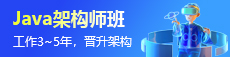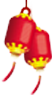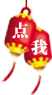#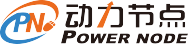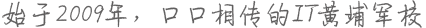### 实现多线程原子性操作

1.基本类型的实现：

package concurrent;

import java.util.concurrent.ExecutorService;

import java.util.concurrent.Executors;

import java.util.concurrent.atomic.AtomicInteger;

public class AtomicTest {

private static final AtomicInteger at=new AtomicInteger();

public static void main(String[] args) {

// TODO Auto-generated method stub

long start=System.currentTimeMillis();

for(int i=0;i<1000;i++)

{

public void run()

{

}

};

es.execute(t1);

}

try

{

}

catch(InterruptedException e)

{

e.printStackTrace();

}

System.out.println("计算过程的耗时为："+(System.currentTimeMillis()-start-5000));

System.out.println("累加1000次，得到结果"+at);

}

}

2.数组类型

package concurrent;

import java.util.concurrent.ExecutorService;

import java.util.concurrent.Executors;

import java.util.concurrent.atomic.AtomicIntegerArray;

public class AtomicTest {

private static final AtomicIntegerArray at=new AtomicIntegerArray(new int);

public static void main(String[] args) {

// TODO Auto-generated method stub

long start=System.currentTimeMillis();

for(int i=0;i<1000;i++)

{

public void run()

{

for(int i=0;i<5;i++)

}

};

es.execute(t1);

}

try

{

}

catch(InterruptedException e)

{

e.printStackTrace();

}

System.out.println("计算过程的耗时为："+(System.currentTimeMillis()-start-5000));

for(int i=0;i<5;i++)

System.out.println("第"+(i+1)+"个元素累加1000次，得到结果"+at.get(i));

}

}

3.属性原子修改器

package concurrent;

import java.util.concurrent.ExecutorService;

import java.util.concurrent.Executors;

import java.util.concurrent.atomic.AtomicIntegerFieldUpdater;

class Count{

public volatile int number;

}

public class AtomicTest {

private static final AtomicIntegerFieldUpdateraifu=AtomicIntegerFieldUpdater.newUpdater(Count.class, "number");

public static void main(String[] args) {

// TODO Auto-generated method stub

final Count count=new Count();

long start=System.currentTimeMillis();

for(int i=0;i<1000;i++)

{

public void run()

{

}

};

es.execute(t1);

}

try

{

}

catch(InterruptedException e)

{

e.printStackTrace();

}

System.out.println("计算过程的耗时为："+(System.currentTimeMillis()-start-5000));

for(int i=0;i<5;i++)

System.out.println("第"+(i+1)+"个元素累加1000次，得到结果"+count.number);

}

}

4.引用类型

package concurrent;

import java.util.concurrent.ExecutorService;

import java.util.concurrent.Executors;

import java.util.concurrent.atomic.AtomicReference;

class Count{

public int count;

public Count(int count)

{

this.count=count;

}

public String toString()

{

return "这个对象的位置是："+count;

}

}

public class AtomicTest {

private static final AtomicReferencear=new AtomicReference();

public static void main(String[] args) {

// TODO Auto-generated method stub

Count count=new Count(1001);

long start=System.currentTimeMillis();

ar.set(count);

System.out.println("你好，"+ar.get());

Count count1=new Count(1002);

ar.compareAndSet(count, count1);//内存值与count是一样的，所以值更新为count1

System.out.println("我发生了改变："+ar.get());

Count count2=new Count(1003);

ar.compareAndSet(count, count2);//此时内存智为count1，与count不一致，所以无法更新，因此内存值依然为count1

System.out.println("我发生了改变："+ar.get());

}

}